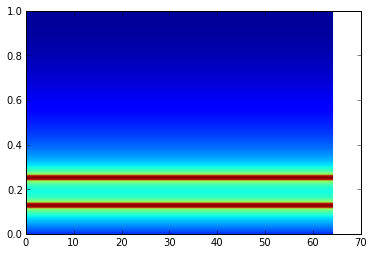# Physical Meaning of a Complex Signal

## Signal

The transmission of communication signals is accomplished by means of a transmission of energy, generally electromagnetic or of acoustic energy. In contrast to the case of power transmission, it isn’t the energy itself which is of interest, but rather the changes in this energy over the course of time. The more complicated the function which represents the change in voltage, current, pressure, or other physical quantity, the greater the amount of information carried by the transmitted energy.

A signal may be considered as being a certain amount of energy, whose distribution in time (given by the waveform of the signal) and in frequency (given by the spectrum) is known. If a signal extends through a distribution of time delta-t and an interval of frequency delta-f, we have a distribution f energy in a rectangle of area delta-t X delt-f

Quadrature signals, also called complex signals, is a two-dimensional signal whose value at some instant in time can be specified as a single complex number having two parts: real part and imaginary part. (The word real and imaginary are unfortunate because of the meaning in every day speech. Communications engineers use the terms in-phase and quadrature phase.)

A signal is a variable (or multiple variables) that changes in time, such as speech or audio signal, or a mesurement like a temperature reading at different hours of a day, or even a stock price that changes over days. A signal can be two dimensional like a video picture. A signal can be continuous (i.e. value can vary continuously and exist for all pints in time) or discrete (i.e. the values exist a discrete points in time and space, typically periodically).

A waveform is the shape and form of a signal such as a wave moving in a physical medium or an abstract representation. In many cases the medium in which the wave is being propagated does not permit a direct visual image of the form. A waveform is an image that represents an audio signal or recording. It shows the changes in amplitude over a certain amount of time. The amplitude of the signal is measured on the y-axis (vertically), while time is measured on the x-axis (horizontally). By extension, the term 'waveform' also describes the shape of the graph of any varying quantity against time.

### Periodic Signals

A sinusoidal signal takes the form $A \cos{\left(2 \pi f_{0} t + \theta \right)}$ where $f_{0} =$ frequency (cycles / second), $T_{0} = 1 / f_0 =$ period, $A =$ amplitude, and $\theta =$ phase (time shift).

Sinusoidal signals are important because they can be used to synthesize any periodic signal. An arbitrary periodic signal can be expressed as a sum of many sinusoidal signals with different frequencies, amplitudes and phases. This repesentation of a periodic signal is called a Fourier Series and takes the form:

A periodic signal has distinct (unique) frequencies. These frequiencies are call harmonics and have a frequency which is some inteiger multiple of the lowest or fundamental fequency ($f_0 = 1/T_0$). Any periodic signal can be approximated by a sum of many sinusoids at harmonic frequencies of the signal ($k f_0$) with appropriate amplitude and phase. The more harmonic components are added, the more accurate the approximation becomes.

Note that we have reduced the representation of any periodic signal to a series of coefficents. Given this infinite series of numbers, you have all that is needed to reproduce the entire function at any moment of time.

### Complex Periodic Signals

Instead of using sinusoidal signals, Euler's formula tells us we can use the complex exponential functions with both positive and negative harmonic frequencies.

### Non-Periodic Signals

A non-periodic arbitrary signal does not have a unique frequency, but can be decomposed into many sinusoidal signals with different frequencies, each with different magnitude and phase.

### Analytic Signals

In mathematics and signal processing, the analytic representation of a real-valued function or signal facilitates many mathematical manipulations of the signal. The basic idea is that the negative frequency components of the Fourier transform (or spectrum) of a real-valued function are superfluous, due to the Hermitian symmetry of such a spectrum. http://en.wikipedia.org/wiki/Analytic_signal

## Fourier Transform

A DFT (Discrete Fourier Transform) is simply the name given to the Fourier Transform when it is applied to digital (discrete) rather than an analog (continuous) signal. An FFT (Fast Fourier Transform) is a faster version of the DFT that can be applied when the number of samples in the signal is a power of two. An FFT computation takes approximately N * log2(N) operations, whereas a DFT takes approximately N^2 operations, so the FFT is significantly faster.

## Physical Meaning of i

Imaginary numbers extent the dimensions of numbers that are otherwise one-dimensional. Its physical existence is that it exists perpendicular to the real line.

There are many ways to view complex numbers, but one of the most intuitive is to think of them as representing points in the plane. Doing so will allow us to interpret basic arithmetic operations like addition and multiplication as performing geometric manipulations, specifically of vectors.

One way to view complex numbers is as a means for converting geometric operations (translation, rotation, scaling) into algebraic operations (adding, multiplication) and back again.

A Visual, Intuitive Guide to Imaginary Numbers - http://betterexplained.com/articles/a-visual-intuitive-guide-to-imaginary-numbers/

Why complex numbers are fundamental in physics - http://motls.blogspot.com/2010/08/why-complex-numbers-are-fundamental-in.html

## Example

Lets make a signal consisting of two Sine waves, with a frequcencies of 1,250 Hz and 625 Hz. We'll represent our signal as 256 values where the sampled values are taken at 10,000 Hz (i.e. 10K samples per second).

In :
x = arange(256.0)
sin1 = sin(2 * pi * (1250.0 / 10000.0) * x)
sin2 = sin(2 * pi * (625.0 / 10000.0) * x)

signal = sin1 + sin2
plot(signal)

Out:
[<matplotlib.lines.Line2D at 0xa200d8c>]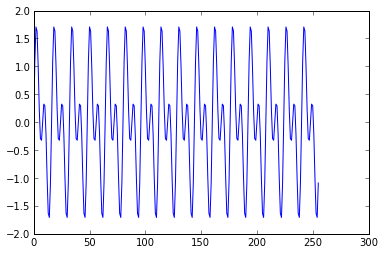To see what this looks like in the frequency domain, we use the Fast Fourier Transform (FFT).

In :
spectrum = fft.rfft(signal)
plot(spectrum)

/usr/lib/python2.7/dist-packages/numpy/core/numeric.py:320: ComplexWarning: Casting complex values to real discards the imaginary part
return array(a, dtype, copy=False, order=order)


Out:
[<matplotlib.lines.Line2D at 0xa25b0ac>]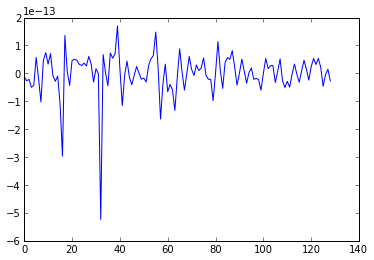Visually, this doesn't look right. We expect two large spikes. Also, we get a warning from iPython, saying we got a complex output. What's happening is that we are ploting only the real part of the complex values.

So lets take the magnitude of the complex output. This give us the following:

In :
plot(abs(spectrum))

Out:
[<matplotlib.lines.Line2D at 0xa5ba4cc>]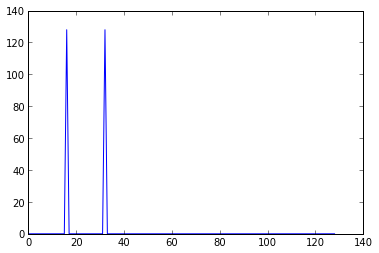A signals energy is given by:

The plot is in raw power output, but generally, we want our power levels in decibels (dB). We can convert this to decibels by:

In :
plot(10 * log10(abs(spectrum)))

Out:
[<matplotlib.lines.Line2D at 0xa60bd0c>]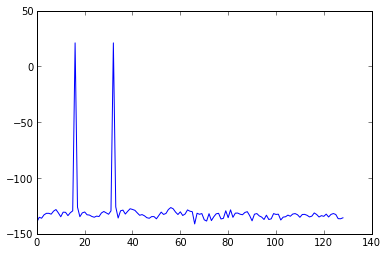So we now see two pure tones, something we expect, but we also have a much lower power signal over a much wider frequency range. Also, we expect the frequency spikes to be at 625 and 1250 Hz. What do the markers on the x-axis represent?

The seamingly random low power singal is really quantization noise.

As to the x-axis, the NumPy FFT function goes from 0 to 5000Hz by default, This means the x-axis markers correspond to values of 0 - 5000 Hz divided into 128 slices. So each marker is 39Hz (5000/128 = 39.0625).

But why 128 slices? Well, 128 is half of the 256 samples and it one-half because the other half are samples along the negative frequency axis, which we are not ploting.

Taking this all into consideration, we can do a more useful plot like:

In []:

Another useful visualization of the frequency domain is the spectrogram. In a spectrogram, time is on the x-axis, frequency is on the y-axis, and amplitude is marked in color. Red is highest amplitude and move through the rainbow of colors to blue , which is the lowest amplitude.

The spectrogram is sort of like extruding the signal out of the screen and looking down upon it from above.

In :
spectgram = specgram(signal)